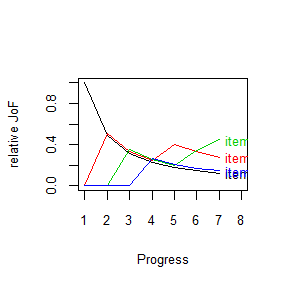# JoF Vignette

## Citation

Burkhardt, M. (2019). JoF: Modelling and Simulating Judgments of Frequency. R package version 0.1.0. https://CRAN.R-project.org/package=JoF

## JoF-package

In a typical experiment for intuitive judgments of frequencies (JoF), different stimuli with different frequencies are presented. To predict or simulate relative judgments of frequency one can use formal models. MINERVA 2 (Hintzman, 1988), TODAM 2 (Murdock, Smith & Bai, 2001), and PASS 1, PASS 2 (both Sedlmeier, 2002) are implemented in the JoF-package.

To simulate an estimation we create four items and a sequence. The items are presented in an abstract way. The value 0 means the absence and the value 1 the presence of a feature. The sequence seq contains the absuolute frequency (e.g. item1 and item4 are presented once, item2 is presented twice and item3 is presented three times)

# create 4 items
item1 <- c(1, 0, 0, 0)
item2 <- c(0, 1, 0, 0)
item3 <- c(0, 0, 1, 0)
item4 <- c(0, 0, 0, 1)
# create a sequence
sqc1 <- c(1, 2, 3, 4, 2, 3, 3)

We also have to specify parameters for attention and decay. These parameters have different implementations and terms (because of the mathematical formulas behind). In this short introduction we show values, which are proven in our simulations (but feel free to try out some new definitions). We give a simple example for PASS 1 and graphing the results over the 7 single presentations.

# Simulation with PASS 1
library(JoF)
set.seed(123)
fit_pass1 <- PASS1(item1, item2, item3, item4,
sqc = sqc1, att = .1, ifc = -.025, dec = -.05,
rdm_weights = FALSE, noise = 0)
fit_pass1
#> relative judgment of frequency
#>
#> item1: 0.125
#> item2: 0.279
#> item3: 0.449
#> item4: 0.146
plot(fit_pass1)The plot give us an impression of the progress of the judgments. If we would brake up the presentation after five Stimuli item 2 would have the highest judgment of frequency.

Now let’s compare the four models for JoF

# Simulation with PASS 2
set.seed(123)
fit_pass2 <- PASS2(item1, item2, item3, item4,
sqc=sqc1, att = .1, n_output_units = "half",
rdm_weights = FALSE, noise = 0)
# Simulation with MINERVA 2
fit_minerva2 <- MINERVA2( item1, item2, item3, item4,
sqc = sqc1, L = 1, dec=NULL)
fit_todam2 <- TODAM2(item1, item2, item3, item4,
sqc = sqc1, gamma = 1, alpha = 1)

matrix(
round(c(fit_pass1$percent_estimate,fit_pass2$percent_estimate,
fit_minerva2$percent_estimate,fit_todam2$percent_estimate),3),
byrow = T, ncol = 4,
dimnames = list(
c("PASS 1", "PASS 2", "MINERVA 2", "TODAM 2"),
c("item1", "item2", "item3", "item4"))
)
#>           item1 item2 item3 item4
#> PASS 1    0.125 0.279 0.449 0.146
#> PASS 2    0.104 0.258 0.462 0.176
#> MINERVA 2 0.143 0.286 0.429 0.143
#> TODAM 2   0.143 0.286 0.429 0.143

MINERVA 2 and TODAM 2 produce perfect estimations because in this example both models work without any error.

Typically, a simulation doesn’t run once. So we can create some more real world conditions (for example random sequence, imperfect learning and decay) and run the simulation 100 times.

# Simulation with 100 replications
set.seed(123)
# PASS 1
p1 <- replicate(100,
{
random_sqc <- sample(sqc1, 7, FALSE)
PASS1(item1, item2, item3, item4,
sqc = random_sqc,
att = .1, ifc = -.025, dec = -.05,
rdm_weights = FALSE,
noise = 0
)$percent_estimate } ) # PASS 2 p2 <- replicate(100, { random_sqc <- sample(sqc1, 7, FALSE) PASS2(item1, item2, item3, item4, sqc = random_sqc, att = .1, n_output_units = "half", rdm_weights = F, noise = 0 )$percent_estimate
}
)
# MINERVA 2
m2 <- replicate(100,
{
random_sqc <- sample(sqc1, 7, FALSE)
MINERVA2(item1, item2, item3, item4,
sqc = random_sqc,
L = .9,
dec = "curve"
)$percent_estimate } ) # TODAM 2 t2 <- replicate(100, { random_sqc <- sample(sqc1, 7, FALSE) TODAM2(item1, item2, item3, item4, sqc = random_sqc, gamma = .9, alpha = .9 )$percent_estimate
}
)
matrix(round(
c(rowMeans(p1), rowMeans(p2), rowMeans(m2), rowMeans(t2)), 3),
ncol = 4, byrow = T,
dimnames = list(c("PASS 1", "PASS 2", "MINERVA 2", "TODAM 2"),
c("Item 1", "Item 2", "Item 3", "Item 4"))
)
#>           Item 1 Item 2 Item 3 Item 4
#> PASS 1     0.146  0.285  0.424  0.146
#> PASS 2     0.146  0.288  0.418  0.148
#> MINERVA 2  0.167  0.280  0.436  0.117
#> TODAM 2    0.142  0.276  0.441  0.141
# theoretical frequancy
table(sqc1)/7
#> sqc1
#>         1         2         3         4
#> 0.1428571 0.2857143 0.4285714 0.1428571

We see slightly differences in judgments of frequency for the models, but all in all, these judgements are quite exact. Remember the correct answer would be 14%; 29%, 43% , 14%. But what we have done in this short demonstration, were extremely simple simulations. We can add variance through another item respresentation. Especially TODAM 2 algorithms have another kind of item representation in the original literature. Also lowering the attention or learning parameters increase variance in estimation. Furthermore, random noise could be added. For simulating empirical results one may think about the conditions of person, environment and the stimulus material and adapt these to the formal model simulations.